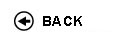Politecnico di Torino
Academic Year 2009/10
11AGIDI, 11AGIEG
Numerical methods
1st degree and Bachelor-level of the Bologna process in Logistics And Production Engineering - Torino
1st degree and Bachelor-level of the Bologna process in Business Organization Engineering - Torino
 Teacher Status SSD Les Ex Lab Tut Years teaching Pieraccini SandraA2 MAT/08 40 0 20 0 7 Pieraccini SandraA2 MAT/08 40 0 20 0 7
 SSD CFU Activities Area context MAT/08 5 C - Affini o integrative Cultura scientifica, umanistica, giuridica, economica, socio-politica
 Objectives of the course The aim is to provide the knowledge for a correct use of scientific libraries for the solution of basic problems. In particular, the attention is focused in the use of MATLAB for solving several kind of problems. Expected skills Knowledge of classical methods for the numerical solution of basic problems. Knowledge of the MATLAB software. Prerequisites Mathematical analysis, geometry Syllabus Floating point arithmetic and its consequences. Classical methods for the numerical solution of some basic problems: linear systems, approximation, numerical integration, ordinary differential equations. Syllabus: more informations Machine numbers and floating-point arithmetic. Error analysis. Condition number. Stability of an algorithm. Solution of systems of linear equations: direct methods and iterative methods. Interpolation and approximation: polynomial interpolation and least square approximation. Numerical integration. Iterative methods for nonlinear equations. One-step methods for ordinary differential equations. Laboratories and/or exercises Classroom: exercises aimed at a deeper comprehension of the topics. Laboratory: in some practical session the students will apply (using MATLAB) the methods introduced during the lectures Bibliography - G. Monegato, Fondamenti di Calcolo Numerico, CLUT Editrice, Torino (1998). - S. Berrone, S. Pieraccini, Esercizi svolti di Calcolo Numerico, con introduzione a MATLAB, CLUT Editrice, Torino (2004). Revisions / Exam Written examination with both practical exercises and theoretical questions concerning all the topics of the program of the course. Programma provvisorio per l'A.A.2006/07© Politecnico di Torino
Corso Duca degli Abruzzi, 24 - 10129 Torino, ITALY